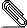[HACKERS] [PATCH] Overestimated filter cost and its mitigation

```Hi hackers,

Currently, cost of a filter with multiple clauses is estimated by
summing up estimated cost of each clause.  As long as a filter
consists of simple clauses and its cost is fairly small, it works
fine. However, when there exists some heavy clauses (like SubQuery or
user-defined functions) and most of tuples are filtered by other
simple clauses, total cost is likely to be overestimated.  An attached
patch mitigates this overestimation by using selectivity estimation of
subsets of clauses in a filter.```
```
Suppose that there are three qual clauses in a scan node, current
postgres estimates per-tuple cost of the filter to be:
cost(A) + cost(B) + cost(C)

And basic idea of the attached patch is:
cost(A) + clauselist_selectivity({A}) * cost(B) +
clauselist_selectivity({A, B}) * cost(C)

We first noticed this overestimation during performance analysis of
our real applications. In order to reproduce the situation, we created
a similar query with pgbench data.

The database was prepared by following commands:
pgbench --scale=1000 -i pgb5
pgbench  -c 10 -t 100000 pgb5

and the query is:
select e.tid,
e.aid,
e.bid,
e.mtime
from pgbench_history e
where e.tid between 1 and 1000
and (select count(*)
from pgbench_history f
where f.mtime < e.mtime
and f.bid = e.bid
group by f.bid) > 100
and e.aid between 1 and 100000;

== Query plan with current upstream ==

1 Seq Scan on pgbench_history e  (cost=0.00..21393523889.00 rows=28
width=20) (actual time=2391.683..21775.191 rows=85 loops=1)
2  Filter: ((tid >= 1) AND (tid <= 1000) AND (aid >= 1) AND (aid <= 100000)
AND ((SubPlan 1) > 100))
3  Rows Removed by Filter: 999915
4    SubPlan 1
5     ->  GroupAggregate  (cost=0.00..21393.50 rows=283 width=12) (actual
time=229.050..229.050 rows=1 loops=94)
6          Group Key: f.bid
7           ->  Seq Scan on pgbench_history f  (cost=0.00..21389.00
rows=333 width=4) (actual time=5.036..228.851 rows=529 loops=94)
8                 Filter: ((mtime < e.mtime) AND (bid = e.bid))
9                 Rows Removed by Filter: 999471

Most amount of total cost 21,393,523,889 comes from:
(cost of SubPlan 1) * (# of rows in pgbench_history) = 21,393.50 *
1,000,000 = 21,393,000,000

but in actual run, SubPlan 1 was executed only 94 times, and total
cost was overestimated more than 10000 times greater.

== Query plan with patched upstream ==

1 Seq Scan on pgbench_history e  (cost=0.00..1687169.88 rows=28 width=20)
(actual time=2388.802..21721.498 rows=85 loops=1)
2   Filter: ((tid >= 1) AND (tid <= 1000) AND (aid >= 1) AND (aid <=
100000) AND ((SubPlan 1) > 100))
3   Rows Removed by Filter: 999915
4   SubPlan 1
5     ->  GroupAggregate  (cost=0.00..19726.83 rows=283 width=12) (actual
time=228.507..228.507 rows=1 loops=94)
6           Group Key: f.bid
7           ->  Seq Scan on pgbench_history f  (cost=0.00..19722.33
rows=333 width=4) (actual time=5.378..228.316 rows=529 loops=94)
8                 Filter: ((mtime < e.mtime) AND (bid = e.bid))
9                 Rows Removed by Filter: 999471

In patched version of upstream, total cost is largely decreased.  In
cost estimation, only 84.4 tuples of pgbench_history are expected to
be evaluated with SubPlan 1.  It is close to actual number 94 to some
extent, and total cost seems much more reasonable than cost with
current upstream.

Any thoughts?

Regards,
Yuto Hayamizu
```Mitigate-filter-cost-overestimation.patch
Description: Binary data

```--
Sent via pgsql-hackers mailing list (pgsql-hackers@postgresql.org)
To make changes to your subscription:
http://www.postgresql.org/mailpref/pgsql-hackers
```
• [HACKERS] [PATCH] Overestimated filter cost and its mitigati... Yuto Hayamizu